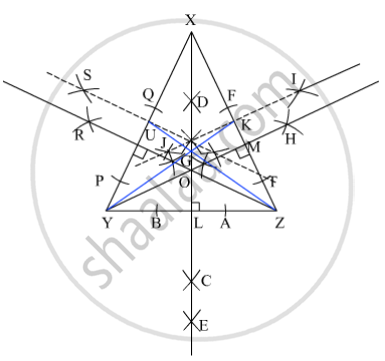# Draw an Isosceles Triangle. Draw All of Its Medians and Altitudes. Write Your Observation About Their Points of Concurrence. - Mathematics

Sum

Draw an isosceles triangle. Draw all of its medians and altitudes. Write your observation about their points of concurrence.

#### SolutionSteps of contruction :
(i) Draw an isosceles ∆XYZ.
(ii) Draw the perpendicular bisector DE of the side YZ that intersect YZ at L.
(iii) Join XL. XL is the median to side YZ.
(iv) With X as centre and taking convenient radius, draw two arcs that intersect YZ at A and B.
(v) With A as centre and taking radius more than half of AB, draw an arc. With B as centre and taking same radius, draw another arc that cut the previous arc at C.
(vi) Join XC that intersect YZ at L. XL is the altitude to the side YZ.
(vii) Draw the perpendicular bisector IJ of the side ZX that intersect ZX at K.
(viii) Join YK. YK is the median to side ZX.
(ix) With Y as centre and taking convenient radius, draw two arcs that intersect ZX at Z and F.
(x) With Z as centre and taking radius more than half of ZF, draw an arc. With F as centre and taking same radius, draw another arc that cut the previous arc at H.
(xi) Join YH that intersect ZX at M. YM is the altitude to the side ZX.
(xii) Draw the perpendicular bisector ST of the side XY that intersect XY at U.
(xiii) Join ZU. ZU is the median to side XY.
(xiv) With Z as centre and taking convenient radius, draw two arcs that intersect XY at P and Q.
(xv) With P as centre and taking radius more than half of PQ, draw an arc. With Q as centre and taking same radius, draw another arc that cut the previous arc at R.
(xvi) Join ZR that intersect XY at N. ZN is the altitude to the side XY.
Hence, ∆XYZ is the required triangle in which the medians XL, YK and ZU to the sides YZ, ZX and XY respectively intersect at G and altitudes XL, YM and ZN to the sides YZ, ZX and XY respectively intersect at O. The point G is the centroid and point O is the orthocentre of ∆XYZ. We observe that, in an isosceles triangle, the point of concurrence of medians (centroid) and altitudes (orthocentre) lie on a same straight line.

Concept: Median of a Triangle
Is there an error in this question or solution?

#### APPEARS IN

Balbharati Mathematics 8th Standard Maharashtra State Board
Chapter 4 Altitudes and Medians of a triangle
Practice Set 4.1 | Q 6 | Page 22